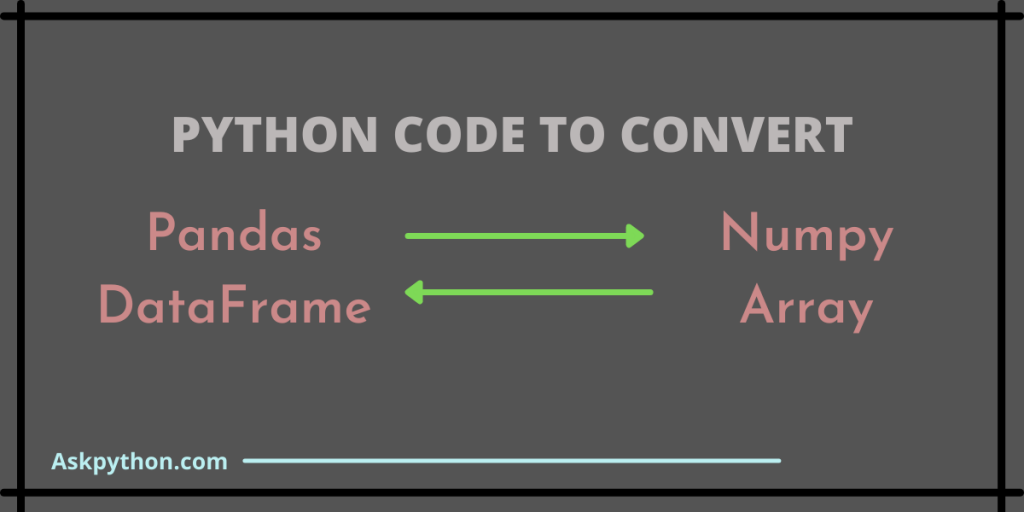# Converting Pandas DataFrame to Numpy Array [Step-By-Step]Hello Reader! In this article, we will see what the data frame is and how to convert Pandas Dataframe to Numpy Array and vice versa. So Let’s begin:

## Introduction

A data frame in Python is a two-dimensional, tabular data structure consisting of rows and columns defining different features of the data frame.

We can create a data frame using the Pandas library or we can import an already built data frame (.csv file) and work on it. You can install Pandas using the pip command.

```pip install pandas
```

The above-written code installs pandas and we are all ready to use different functions of the Pandas library. In the same way, we will install the numpy library:

```pip install numpy
```

So first, we will see the conversion of this tabular structure (pandas data frame) into a numpy array.

### 1. Converting Pandas Dataframe to Numpy Array

We can do this by using dataframe.to_numpy() method. This will convert the given Pandas Dataframe to Numpy Array.

• Let us create two data frames which we will be using for this tutorial.
```#importing pandas
import pandas as pd

#creating dataframes
student_data = {"Name": ['Alice', 'Sam', 'Kevin', 'Max', 'Tom'],
"exam_no": [201, 202, 203, 204, 205],
"Result": ['Pass', 'Pass', 'Fail', 'Pass', 'Fail']}

set_of_numbers = {"Numbers": ['134', '273', '325','69.21','965']}

print("This is our first dataset :")
student_dataframe = pd.DataFrame(student_data)
print("\n",student_dataframe)

print("\nThis is our second dataset :")
numbers_dataframe = pd.DataFrame(set_of_numbers)
print("\n",numbers_dataframe)
```
• We have created two data frames: student_data and set_of_numbers. Our data frames look like this:
• Now, before converting the Pandas Dataframe to Numpy Array, let’s see the type :
```print(type(student_dataframe))
```
```print(type(numbers_dataframe))
```

The output for both the statements above is the same. I.e.,

```<class 'pandas.core.frame.DataFrame'>
```
• To convert this Pandas Dataframe to Numpy Array, run the code given below

Converting student_data to

```student_array = student_dataframe.to_numpy()
print(student_array)
```

Output :

```[['Alice' 201 'Pass']
['Sam' 202 'Pass']
['Kevin' 203 'Fail']
['Max' 204 'Pass']
['Tom' 205 'Fail']]
```

For the second data frame (set_of_numbers)

```numbers_array = numbers_dataframe.to_numpy()
print(numbers_array)
```

Output :

```[['134']
['273']
['325']
['69.21']
['965']]
```
• We can also check the datatypes for both the arrays :
```print(type(student_array))
print(type(numbers_array))
```

Output :

```<class 'numpy.ndarray'>
<class 'numpy.ndarray'>
```

So, we can clearly see that we converted our Pandas Dataframe to Numpy Array in just a few steps. This is the simplest way to handle data frames and their conversion.

• Further, we can also change the data type of columns in a data frame. Considering our second data frame, it consists of some integer values and some floating values, let’s try to change all of them to float.
```print(numbers_dataframe.to_numpy(dtype ='float64'))
```

Output :

```[[134.  ]
[273.  ]
[325.  ]
[ 69.21]
[965.  ]]
```

### 2. Converting Numpy Arrays to Pandas Dataframes

Now that you have understood the conversion of the Pandas Dataframe to Numpy Array, we may need to convert the data back to Numpy Array. Let’s see how to do that:

• First, define a numpy array. And then perform the conversion using pandas.DataFrame() function of pandas library.
```#importing pandas and numpy
import pandas as pd
import numpy as np

#defining numpy array
arr1 = np.array([[1,6,4,5], [3,7,2,4], [9,5,3,7]])
print("Numpy array : ")
print(arr1)
```

So, our array is like this:

```Numpy array :
[[1 6 4 5]
[3 7 2 4]
[9 5 3 7]]
```
• Now, converting this to pandas dataframe:
```#converting array to dataframe
df = pd.DataFrame(arr1)
print("\npandas dataframe :")
df
```

The converted data frame is :

• Checking the type of dataframe:
```type(df)
```

Output:

```pandas.core.frame.DataFrame
```
• We can also give our own headers to rows and columns of the data frames. Headers for rows can be given using index keyword and, for columns, we use the columns keyword.
```#converting and providing headers
df = pd.DataFrame(arr1, index = ["1","2","3"], columns = ["A","B","C","D" ])
print("\npandas dataframe :")
df
```

This will make our data frame look like this :